DOI: https://doi.org/10.20535/1810-0546.2018.5.147067

### Amplitude-Frequency Characteristics of the System “Mixing–Mixing” Heat Exchanger + Heat Transfer”

Igor D. Lucheyko, Roman V. Kotsiurko

#### Abstract

Background. Mathematical modeling of continuous technological processes in non-stationary conditions caused by actions of various destabilizing factors is an actual problem. At the same time, analytical solutions have significant advantages over numerical ones, since they allow us to find out the nature of causation links in the analyzed properties of the modeling object and, as a practical result, to give physically well-founded recommendations for improving the efficiency of its functioning. The possible fluctuations in the temperature of heat carrier (HC) at the inputs of the flow heat exchanger (HE) cause instabilities for heat exchange processes. This usually leads to the efficiency decrease of the HE. Therefore, the calculation of its amplitude-frequency characteristics (AFC) has a significant (weighty) value.

Objective. The aim of the paper is to find out the behavior of the ideal “mixing–mixing” HE in stationary mode and harmonic fluctuations of temperature at the inputs of the apparatus and calculation of its AFC.

Methods. Known mathematical model in the form of a system of linear differential equations (reduced summed to dimensionless form) to calculate the effect of harmonic fluctuations of temperature on the stationary mode of the system “HE + heat transfer” was used.

Results. It is shown that the efficiency of the stationary mode of operation of the ideal “mixing – mixing” HE can be estimated by the efficiency indicator, where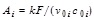– transfer number (k – heat transfer coefficient across the surface area F– the volumetric flow rates of HC and their heat capacities). When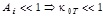, when(the process of heat transfer is much more intense compared with the process of heat outlet by flows from HE). AFCof the system is calculated, where– the simplex of the amplitudes of temperature fluctuations at the inputs and outputs;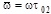– frequency complex (w – cyclic frequency, t02 – average time of stay of cold HC in HE).

Conclusions. In the case of common-phase fluctuations of temperatures at the inputs of HE AFC don’t depend on Ai, i.e. they are equal to the AFC of HE as two flow devices of the ideal mixing. In the case of antiphase fluctuations of temperature the values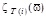are equal when the formal rearrangement of values Ai:, which is a reflection of the thermal “equality” of HC. When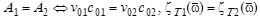; when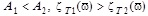at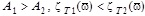. In the case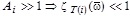(the intense heat transfer reduces the amplitudes of temperature fluctuations at the outputs of the HC). At relatively high frequencies, stationary mode is practically not violated at any values of Ai:. Therefore, in order to ensure practically stationary mode of HE operation, it is necessary to get rid of low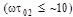harmonics of possible temperature fluctuations at the inputs.

#### Keywords

Mathematical modeling; “Mixing–mixing” heat exchanger; Performance indicator; Harmonic temperature fluctuations; Amplitude-frequency characteristic

PDF (Українська)

#### References

W.M. Kays and A.L. London, Compact Heat Exchangers. Florida, Malabar: Krieger Publishing Company, 1998.

A.G. Bondar, Mathematical Modeling in Chemical Technology. Kyiv, Ukraine: Vyshcha Shkola, 1973.

H. Hausen, Heat Transfer in Countercurrent, Concurrent and Crosscurrent Flow. Heidelberg, Germany: Springer Verlag, 1976.

W.L. Luyben, Process Modeling, Simulation, and Control for Chemical Engineers. New York: McGraw-Hill, 1996.

J.M. Coulson and J.F. Richardson, Chemical Engineering, vol. 1, Fluid Flow, Heat Transfer and Mass Transfer. Oxford: Butterworth-Heinemann, 1999.

A.D. Polyanin et al., Hydrodynamics, Mass and Heat Transfer in Chemical Engineering. London: Gordon and Breach Sci. Publ., 2002.

C. Boyadjiev, Theoretical Chemical Engineering. Modeling and Simulation. Berlin, Heidelberg, Germany: Springer-Verlag, 2010.

A.K Jana, Chemical Process Modelling and Computer Simulation. Delhi: PHI Learning Private Limited, 2011.

A. Rasmuson et al., Mathematical Modeling in Chemical Engineering. Cambridge: United Kingdom by MPG Print-group Ltd, 2014.

R. Serthn and T. Lestina, Process Heat Transfer. Principles and Applications. Cambridge, UK: Academic Press, Elsevier, 2014.

J.R. Welty et al., Fundamentals of Momentum, Heat, and Mass Transfer. New York: John Wiley & Sons Inc., 2014.

J.-P. Duroudier, Heat Transfer in the Chemical, Food and Pharmaceutical Industries. London, UK: ISTE Press, 2016.

I.D. Lucheyko and R.V. Kotsiurko, “Concurrent and countercurrent flow mode comparison in the tube in tube heat exchanger”, Naukovyi Ogliad, vol. 28, no. 7, pp. 15–26, 2016.

I.D. Lucheyko and R.V. Kotsiurko, “Efficiency coefficient of the “tube in tube” heat exchanger in the concurrent flow”, in Proc. XX Mendeleev Congress on General and Applied Chemistry, vol. 3, Yekaterinburg, Russia, 2016.

I.D. Lucheyko and R.V. Kotsiurko, “Temperature efficiency coefficient of the “tube in tube” heat exchanger in the countercurrent flow”, in Proc. XVІ Int. Sci. Conf. “High-Tech in Chemical Engineering–2016, Moscow, Russia, 2016.

R.V. Kotsiurko and I.D. Lucheyko, “Mixing-mixing” heat exchanger as temperature signal transducer”, in Proc. VІ Int. Sci. Tech. Conf. “Current Issues in Modern Technologies”, Ternopil, Ukraine, 2017.

I.D. Lucheyko et al., “Frequency characteristics of a continuous stirred tank reactor at small perturbations of the concentration of a reagent (reaction )”, Visnyk TDTU im. I. Puliuja, vol. 11, no. 3, pp. 195–204, 2006.

#### GOST Style Citations

Copyright (c) 2018 Igor Sikorsky Kyiv Polytechnic Institute